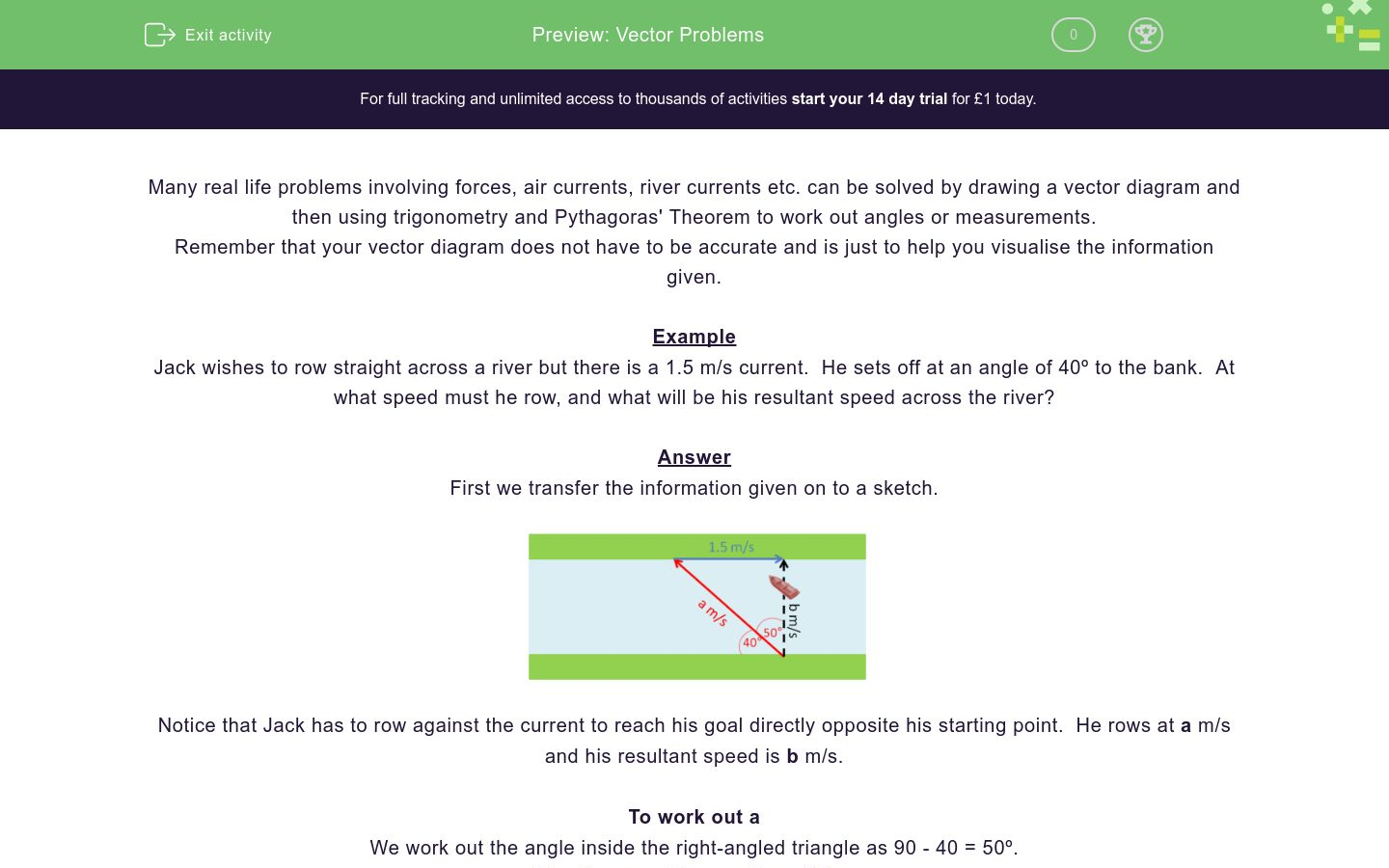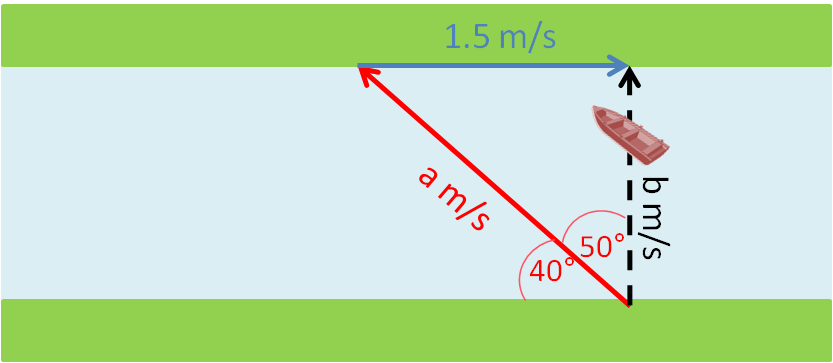# Vector Problems

In this worksheet, students use vector diagrams, trigonometry and Pythagoras' Theorem to answer real life problems.Key stage:  KS 4

Curriculum topic:  Geometry and Measures

Difficulty level:### QUESTION 1 of 10

Many real life problems involving forces, air currents, river currents etc. can be solved by drawing a vector diagram and then using trigonometry and Pythagoras' Theorem to work out angles or measurements.

Remember that your vector diagram does not have to be accurate and is just to help you visualise the information given.

Example

Jack wishes to row straight across a river but there is a 1.5 m/s current.  He sets off at an angle of 40º to the bank.  At what speed must he row, and what will be his resultant speed across the river?

Answer

First we transfer the information given on to a sketch.Notice that Jack has to row against the current to reach his goal directly opposite his starting point.  He rows at a m/s and his resultant speed is b m/s.

To work out a

We work out the angle inside the right-angled triangle as 90 - 40 = 50º.

The trigonometric equation will be:

1.5/a = sin 50º

a = 1.5/sin 50º

a = 1.96 m/s

To work out b

Method 1

The trigonometric equation will be:

1.5/b = tan 50º

b = 1.5/tan 50º

b = 1.26 m/s

Method 2

Pythaoras' Equation will be:

1.52 + b2 = a2

b2 = a2 - 1.52 = 1.962 - 1.52 = 1.5916

b = √1.5916 = 1.26 m/s

Jill wishes to row straight across a river but there is a 2 m/s current.  She sets off at an angle of 50º to the bank.  At what speed must she row in m/s to 3 sig. figs?

(just write the number)Jill wishes to row straight across a river but there is a 2 m/s current.  She sets off at an angle of 50º to the bank.  What will be her resultant speed across the river in m/s to 3 sig. figs?

(just write the number)Ahmed wishes to row straight across a river but there is a 2 m/s current.  He rows off at at a speed of 3m/s.  At what angle to the bank, to the nearest degree, must he set off in order to cross to a point directly opposite on the other bank?

(just write the number)Ahmed wishes to row straight across a river but there is a 2 m/s current.  He rows off at at a speed of 3m/s.  What will his resultant speed be in m/s to 3 sig figs?

(just write the number)The pilot of a plane wishes to fly due North but there is a 60mph Easterly wind (i.e. from the East.  The plane's airspeed is 500 mph.  At what bearing must the pilot fly the plane in order to head due North?

(just write the three figure number)The pilot of a plane wishes to fly due North but there is a 60mph Easterly wind (i.e. from the East.  The plane's airspeed is 500 mph.  What will be the resultant speed in mph of the plane across the ground?

(just write the number to 3 sig figs)The pilot of a plane wishes to fly due North but there is an 80mph Easterly wind (i.e. from the East.  The plane's airspeed is 200 mph.  At what bearing must the pilot fly the plane in order to head due North?

(just write the three figure number)The pilot of a plane wishes to fly due North but there is an 80mph Easterly wind (i.e. from the East.  The plane's airspeed is 200 mph.  What will be the resultant speed in mph of the plane across the ground?

(just write the number to 3 sig figs)The pilot of a plane wishes to fly due North but there is a 75mph Easterly wind (i.e. from the East.  The plane flies on a bearing of 035º.  At what airspeed in mph must the pilot fly in order to maintain a northerly heading across the ground?

(just write the number to 3 sig figs)The pilot of a plane wishes to fly due North but there is a 75mph Easterly wind (i.e. from the East.  The plane flies on a bearing of 035º.  What will be the resultant speed in mph across the ground in a northerly direction?

(just write the number to 3 sig figs)• Question 1

Jill wishes to row straight across a river but there is a 2 m/s current.  She sets off at an angle of 50º to the bank.  At what speed must she row in m/s to 3 sig. figs?

(just write the number)CORRECT ANSWER
3.11
EDDIE SAYS
2/a = sin40°
a = 2 ÷ sin40°
• Question 2

Jill wishes to row straight across a river but there is a 2 m/s current.  She sets off at an angle of 50º to the bank.  What will be her resultant speed across the river in m/s to 3 sig. figs?

(just write the number)CORRECT ANSWER
2.38
EDDIE SAYS
2/b = tan40°
b = 2 ÷ tan40°
• Question 3

Ahmed wishes to row straight across a river but there is a 2 m/s current.  He rows off at at a speed of 3m/s.  At what angle to the bank, to the nearest degree, must he set off in order to cross to a point directly opposite on the other bank?

(just write the number)CORRECT ANSWER
48
EDDIE SAYS
The angle at the top left of the triangle is alternate and therefore equal to θ°
2/3 = cosθ°
θ° = cos-1(2/3)
• Question 4

Ahmed wishes to row straight across a river but there is a 2 m/s current.  He rows off at at a speed of 3m/s.  What will his resultant speed be in m/s to 3 sig figs?

(just write the number)CORRECT ANSWER
2.24
EDDIE SAYS
Using Pythagoras' Theorem, b² = 3² - 2²
b = √5
• Question 5

The pilot of a plane wishes to fly due North but there is a 60mph Easterly wind (i.e. from the East.  The plane's airspeed is 500 mph.  At what bearing must the pilot fly the plane in order to head due North?

(just write the three figure number)CORRECT ANSWER
007
EDDIE SAYS
sin θ° = 60/500 = 0.12
θ° = sin-10.12 = 6.89
Bearing has three digits
• Question 6

The pilot of a plane wishes to fly due North but there is a 60mph Easterly wind (i.e. from the East.  The plane's airspeed is 500 mph.  What will be the resultant speed in mph of the plane across the ground?

(just write the number to 3 sig figs)CORRECT ANSWER
496
EDDIE SAYS
Using Pythagoras' Theorem, b² = 500² - 60²
b = √246400
• Question 7

The pilot of a plane wishes to fly due North but there is an 80mph Easterly wind (i.e. from the East.  The plane's airspeed is 200 mph.  At what bearing must the pilot fly the plane in order to head due North?

(just write the three figure number)CORRECT ANSWER
024
EDDIE SAYS
sin θ° = 80/200 = 0.4
θ° = sin-10.4 = 23.6
Bearing has three digits
• Question 8

The pilot of a plane wishes to fly due North but there is an 80mph Easterly wind (i.e. from the East.  The plane's airspeed is 200 mph.  What will be the resultant speed in mph of the plane across the ground?

(just write the number to 3 sig figs)CORRECT ANSWER
183
EDDIE SAYS
Using Pythagoras' Theorem, b² = 200² - 80²
b = √33600
• Question 9

The pilot of a plane wishes to fly due North but there is a 75mph Easterly wind (i.e. from the East.  The plane flies on a bearing of 035º.  At what airspeed in mph must the pilot fly in order to maintain a northerly heading across the ground?

(just write the number to 3 sig figs)CORRECT ANSWER
131
EDDIE SAYS
sin 35° = 75/a
a = 75 ÷ sin 35°
• Question 10

The pilot of a plane wishes to fly due North but there is a 75mph Easterly wind (i.e. from the East.  The plane flies on a bearing of 035º.  What will be the resultant speed in mph across the ground in a northerly direction?

(just write the number to 3 sig figs)CORRECT ANSWER
107
EDDIE SAYS
Using Pythagoras' Theorem, b² = 131² - 75²
b = √11536
or b = 75 ÷ tan 35°
---- OR ----

Sign up for a £1 trial so you can track and measure your child's progress on this activity.

### What is EdPlace?

We're your National Curriculum aligned online education content provider helping each child succeed in English, maths and science from year 1 to GCSE. With an EdPlace account you’ll be able to track and measure progress, helping each child achieve their best. We build confidence and attainment by personalising each child’s learning at a level that suits them.

Start your £1 trial